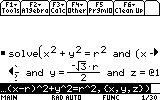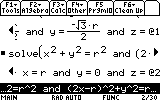# Knowledge Base

## Solution 24995: Answers that Include an @1 or a Component Formatted as @k on the TI-89 Family, TI-92 Family, or Voyage™ 200

### What does an @1 or a component formatted as @k in the answer on the TI-89 Family, TI-92 family, or Voyage 200 mean?

The appearance of @1 in an answer means that the answer is true for any constant that replaces @1.  Constants are represented by the format @k, where @ is any constant and k represents another iteration of the constant and can be between 1 and 255.

The example below shows that the first time a constant appears in an answer it is listed as @1, with the command solve(x^2+y^2=r^2 and (x-r)^2+y^2=r^2,{x,y,z}) and the answer as x=r/2 and y=(square root of 3*r)/2 and z=@1 or x=r/2 and y=-(square root of 3*r)/2 and z=@1.The next example shows that the second appearance of the constant representation, in the same or another problem, is listed as @2 and the next iterations will show as @3,@4....@k.  The equation below is a slight variation on the command above: solve(x^2+y^2=r^2 and (2x-r)^2+y^2=r^2,{x,y,z}) and the answer is x=r/3 and y=(2*square root of 2*r)/3 and z=@2 or x=r/3 and y=(-2*square root of 2*r)/3 and z=@2 or x=r and y=0 and z=@2.Please see the TI-89 family, TI-92 family and Voyage 200 guidebooks for additional information.